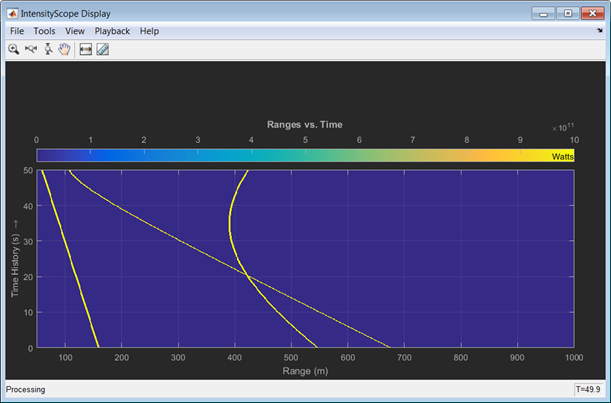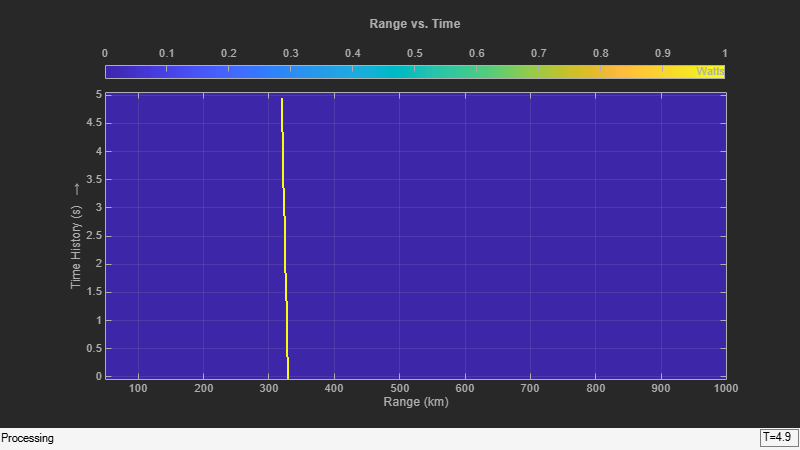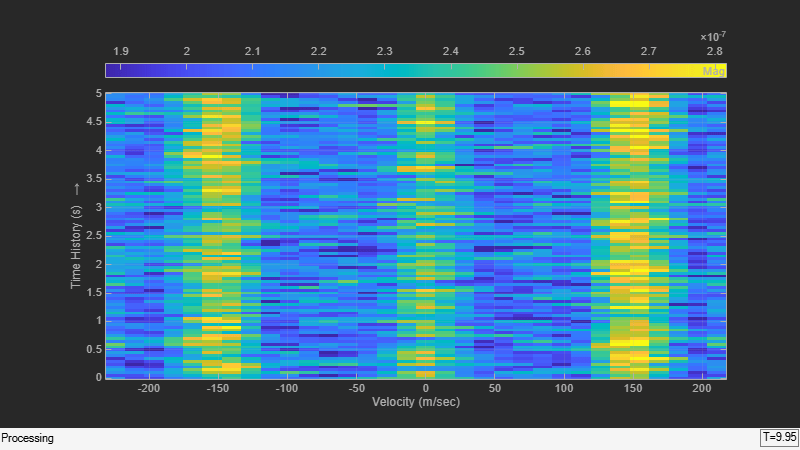# phased.IntensityScope

Range-time-intensity (RTI) or Doppler-time-intensity (DTI) display

## Description

The `phased.IntensityScope` System object™ creates an intensity scope for viewing range-time-intensity (RTI) or Doppler-time-intensity (DTI) data. An intensity scope is a scrolling waterfall of intensity values as a function of time. Scan lines appear at the bottom of the display window and scroll off at the top. Each scan line represents signal intensity as a function of a parameter of interest, such as range or speed. You can also use this object to display angle-time-intensity data and spectral data. This figure shows an RTI display.To create an intensity scope:

1. Define and set up the `phased.IntensityScope` System object. You can set any System object properties at this time or you can leave them at their default values. See Construction .

2. Call the `step` method to add intensity lines to the bottom of the display according to the properties of the `phased.IntensityScope` System object. Some properties are tunable and can be changed at any time. Non-tunable properties cannot be changed after the first call to `step`. Subsequent calls to `step` add more intensity lines.

Note

Starting in R2016b, instead of using the `step` method to perform the operation defined by the System object, you can call the object with arguments, as if it were a function. For example, `y = step(obj,x)` and `y = obj(x)` perform equivalent operations.

## Construction

`sIS = phased.IntensityScope` creates an intensity scope System object, `sIS`, having default property values.

`sIS = phased.IntensityScope(Name,Value)` returns an intensity scope System object, `sIS`, with each specified property `Name` set to a specified `Value`. `Name` must appear inside single quotes (`''`). You can specify several name-value pair arguments in any order as `Name1,Value1,...,NameN,ValueN`.

## Properties

expand all

Intensity scope window name, specified as a character vector. `Name` property and `Title` are different properties. The title appears inside the display window, above the data. The name appears in the title bar of the window.

Example: `'Range Intensity'`

Data Types: `char`

X-axis sample spacing, specified as a positive real-valued scalar. This quantity determines the width of each horizontal bin of the scan line. The units depend on the interpretation of the data. For example, if you are creating an RTI display, then setting `XResolution` to `0.5` is interpreted as 0.5 meters.

Example: `0.5`

Data Types: `double`

X-axis offset, specified as a real-valued scalar. This quantity sets the value of the lowest bin of the scan line. The values of all other bins are equal to this value plus an integer multiple of `Xresolution`. The units depend upon the interpretation of the data. For example, if you are creating an RTI display, then setting `XOffset` to `100.0` is interpreted as 100 meters.

Example: `-0.1`

Data Types: `double`

X-axis label, specified as a character vector.

Example: `'Range (km)'`

Data Types: `char`

Title of the intensity scope display, specified as a character vector. `Title` property and `Name` are different properties. The title appears inside the display window, above the data. The name appears in the title bar of the window.

Example: `'Range vs Time'`

Data Types: `char`

Time resolution of intensity line(s), specified as a positive real-valued scalar. Units are seconds.

Example: `.0001`

Data Types: `double`

Time span of intensity display, specified as a positive real-valued scalar. Units are seconds.

Example: `5.0`

Data Types: `double`

Intensity units label displayed in the color bar, specified as a character vector.

Example: `'Watts'`

Data Types: `char`

Location and size of the intensity scope window, specified as a 1-by-4 vector having the form `[left bottom width height]`. Units are in pixels.

• `left` and `bottom` specify the location of the bottom-left corner of the window.

• `width` and `height` specify the width and height of the window.

The default value of this property depends on the resolution of your display. This property is tunable.

Example: `[100 100 500 400]`

Data Types: `double`

## Methods

 hide Hide intensity scope window reset Reset state of intensity scope System object show Show intensity scope window step Update intensity scope display
Common to All System Objects
`release`

Allow System object property value changes

## Examples

collapse all

Use a `phased.IntensityScope` System object™ to display the echo intensity of a moving target as a function of range and time.

Run the simulation for 5 seconds at 0.1 second steps. In the display, each horizontal scan line shows the intensities of radar echo at each time step.

```nsteps = 50; dt = .1; timespan = nsteps*dt; ```

Simulate a target at a range of 320.0 km and a range rate of 2.0 km/s. Echoes are resolved into range bins of 1 km resolution. The range bins span from 50 to 1000 km.

```rngres = 1.0; rngmin = 50.0; rngmax = 1000.0; tgtrange = 320.0; rangerate = 2.0; rngscan = [rngmin:rngres:rngmax]; ```

Set up the Intensity Scope using these properties.

• Use the `XResolution` property to set the width of each scan line bin to the range resolution of 1 km.

• Use the `XOffset` property to set the value of the lowest range bin to the minimum range of 50 km.

• Use the `TimeResolution` property to set the value of the scan line time difference to 0.1 s.

• Use the `TimeSpan` property to set the height of the display window to the time duration of the simulation.

• Use the `IntensityUnits` property to set the display units to `Watts`.

```scope = phased.IntensityScope('Name','IntensityScope Display',... 'Title','Range vs. Time','XLabel','Range (km)',... 'XResolution',rngres,'XOffset',rngmin,... 'TimeResolution',dt,'TimeSpan',timespan, ... 'IntensityUnits','Watts','Position',[100,100,800,450]); ```

Update the current target bin and create entries for two adjacent range bins. Each call to the `step` method creates a new scan line.

```for k = 1:nsteps bin = floor((tgtrange - rngmin)/rngres) + 1; scanline = zeros(size(rngscan)); scanline(bin+[-1:1]) = 1; scope(scanline.'); tgtrange = tgtrange + dt*rangerate; pause(.1); end ```Use the `phased.IntensityScope` System object™ to display the intensities of the echoes of three moving targets as functions of range and time.

Create the Radar and Target System Objects

Set up the initial positions and velocities of the three targets. Use the `phased.Platform` System object to model radar and target motions. The radar is stationary while the targets undergo constant velocity motion. The simulation runs for 500 steps at 0.1 second increments, giving a total simulation time of 50 seconds.

```nsteps = 500; dt = .1; timespan = nsteps*dt; x1 = [60,0,0]'; x2 = [60,-80,40]'; x3 = [300,0,-300]'; v1 = [2,0,0]'; v2 = [10,5,6]'; v3 = [-10,2,-4]'; platform = phased.Platform([0,0,0]',[0,0,0]'); targets = phased.Platform([x1,x2,x3],[v1,v2,v3]); ```

Set Up Range Bins

Each echo is put into a range bin. The range bin resolution is 1 meter and the range is from 50 to 1000 meters.

```rngres = 1.0; rngmin = 50.0; rngmax = 1000.0; rngscan = [rngmin:rngres:rngmax]; ```

Create the Gain Function

Define a range-dependent gain function to enhance the display of targets at larger ranges. The gain function amplifies the returned echo for visualization purposes only.

```rangegain = @(rng)(1e12*rng^4); ```

Create the Intensity Scope

Set up the Intensity Scope using these properties.

• Use the `XResolution` property to set the width of each scan line bin to the range resolution of 1 km.

• Use the `XOffset` property to set the value of the lowest range bin to the minimum range of 50 km.

• Use the `TimeResolution` property to set the value of the scan line time difference to 0.1 s.

• Use the `TimeSpan` property to set the height of the display window to the time duration of the simulation.

• Use the `IntensityUnits` property to set the display units to `Watts`.

```scope = phased.IntensityScope('Name','IntensityScope Display',... 'Title','Ranges vs. Time','XLabel','Range (m)','XResolution',rngres,... 'XOffset',rngmin,'TimeResolution',dt,'TimeSpan',timespan, ... 'IntensityUnits','Watts','Position',[100,100,800,450]); ```

Run Simulation Loop

1. In this loop, move the targets at constant velocity using the `step` method of the `phased.Platform` System object.

2. Compute the target ranges using the `rangeangle` function.

3. Compute the target range bins by quantizing the range values in integer multiples of `rngres`.

4. Fill each target range bin and neighboring bins with a simulated radar intensity value.

5. Add the signal from each target to the scan line.

6. Call the `step` method of the `phased.IntensityScope` System object to display the scan lines.

```for k = 1:nsteps xradar = platform(dt); xtgts = targets(dt); [rngs] = rangeangle(xtgts,xradar); scanline = zeros(size(rngscan)); rngindx = ceil((rngs(1) - rngmin)/rngres); scanline(rngindx + [-1:1]) = rangegain(rngs(1))/(rngs(1)^4); rngindx = ceil((rngs(2) - rngmin)/rngres); scanline(rngindx + [-1:1]) = rangegain(rngs(2))/(rngs(2)^4); rngindx = ceil((rngs(3) - rngmin)/rngres); scanline(rngindx + [-1:1]) = rangegain(rngs(3))/(rngs(3)^4); scope(scanline.'); pause(.1); end ```Use the `phased.IntensityScope` System Object™ to display the detection output of a radar system simulation. The radar scenario contains a stationary single-element monostatic radar and three moving targets.

Set Radar Operating Parameters

Set the maximum range, peak power range resolution, operating frequency, transmitter gain, and target radar cross-section.

```max_range = 5000; range_res = 50; fc = 10e9; tx_gain = 20; peak_power = 5500.0; ```

Choose the signal propagation speed to be the speed of light, and compute the signal wavelength corresponding to the operating frequency.

```c = physconst('LightSpeed'); lambda = c/fc; ```

Compute the pulse bandwidth from the range resolution. Set the sampling rate, `fs`, to twice the pulse bandwidth. The noise bandwidth is also set to the pulse bandwidth. The radar integrates a number of pulses set by `num_pulse_int`. The duration of each pulse is the inverse of the pulse bandwidth.

```pulse_bw = c/(2*range_res); pulse_length = 1/pulse_bw; fs = 2*pulse_bw; noise_bw = pulse_bw; num_pulse_int = 10; ```

Set the pulse repetition frequency to match the maximum range of the radar.

```prf = c/(2*max_range); ```

Create System Objects for the Model

Choose a rectangular waveform.

```waveform = phased.RectangularWaveform('PulseWidth',pulse_length,... 'PRF',prf,'SampleRate',fs); ```

Set the receiver amplifier characteristics.

```amplifier = phased.ReceiverPreamp('Gain',20,'NoiseFigure',0,... 'SampleRate',fs,'EnableInputPort',true,'SeedSource','Property',... 'Seed',2007); transmitter = phased.Transmitter('Gain',tx_gain,'PeakPower',peak_power,... 'InUseOutputPort',true); ```

Specify the radar antenna as a single isotropic antenna.

```antenna = phased.IsotropicAntennaElement('FrequencyRange',[5e9 15e9]); ```

Set up a monostatic radar platform.

```radarplatform = phased.Platform('InitialPosition',[0; 0; 0],... 'Velocity',[0; 0; 0]); ```

Set up the three target platforms using a single System object.

```targetplatforms = phased.Platform(... 'InitialPosition',[2000.66 3532.63 3845.04; 0 0 0; 0 0 0], ... 'Velocity',[150 -150 0; 0 0 0; 0 0 0]); ```

Create the radiator and collector System objects.

```radiator = phased.Radiator('Sensor',antenna,'OperatingFrequency',fc); collector = phased.Collector('Sensor',antenna,'OperatingFrequency',fc); ```

Set up the three target RCS properties.

```targets = phased.RadarTarget('MeanRCS',[1.6 2.2 1.05],'OperatingFrequency',fc); ```

Create System object to model two-way freespace propagation.

```channels= phased.FreeSpace('SampleRate',fs,'TwoWayPropagation',true,... 'OperatingFrequency',fc); ```

Define a matched filter.

```MFcoef = getMatchedFilter(waveform); filter = phased.MatchedFilter('Coefficients',MFcoef,'GainOutputPort',true); ```

Create Range and Doppler Bins

Set up the fast-time grid. Fast time is the sampling time of the echoed pulse relative to the pulse transmission time. The range bins are the ranges corresponding to each bin of the fast time grid.

```fast_time = unigrid(0,1/fs,1/prf,'[)'); range_bins = c*fast_time/2; ```

To compensate for range loss, create a time varying gain System Object.

```gain = phased.TimeVaryingGain('RangeLoss',2*fspl(range_bins,lambda),... 'ReferenceLoss',2*fspl(max_range,lambda)); ```

Set up Doppler bins. Doppler bins are determined by the pulse repetition frequency. Create an FFT System object for Doppler processing.

```DopplerFFTbins = 32; DopplerRes = prf/DopplerFFTbins; fft = dsp.FFT('FFTLengthSource','Property',... 'FFTLength',DopplerFFTbins); ```

Create Data Cube

Set up a reduced data cube. Normally, a data cube has fast-time and slow-time dimensions and the number of sensors. Because the data cube has only one sensor, it is two-dimensional.

```rx_pulses = zeros(numel(fast_time),num_pulse_int); ```

Create IntensityScope System Objects

Create two `IntensityScope` System objects, one for Doppler-time-intensity and the other for range-time-intensity.

```dtiscope = phased.IntensityScope('Name','Doppler-Time Display',... 'XLabel','Velocity (m/sec)', ... 'XResolution',dop2speed(DopplerRes,c/fc)/2, ... 'XOffset',dop2speed(-prf/2,c/fc)/2,... 'TimeResolution',0.05,'TimeSpan',5,'IntensityUnits','Mag'); rtiscope = phased.IntensityScope('Name','Range-Time Display',... 'XLabel','Range (m)', ... 'XResolution',c/(2*fs), ... 'TimeResolution',0.05,'TimeSpan',5,'IntensityUnits','Mag'); ```

Run the Simulation Loop over Multiple Radar Transmissions

Transmit 2000 pulses. Coherently process groups of 10 pulses at a time.

For each pulse:

1. Update the radar position and velocity `radarplatform`

2. Update the target positions and velocities `targetplatforms`

3. Create the pulses of a single wave train to be transmitted `transmitter`

4. Compute the ranges and angles of the targets with respect to the radar

5. Radiate the signals to the targets `radiator`

6. Propagate the pulses to the target and back `channels`

7. Reflect the signals off the target `targets`

8. Receive the signal `sCollector`

9. Amplify the received signal `amplifier`

10. Form data cube

For each set of 10 pulses in the data cube:

1. Match filter each row (fast-time dimension) of the data cube.

2. Compute the Doppler shifts for each row (slow-time dimension) of the data cube.

```pri = 1/prf; nsteps = 200; for k = 1:nsteps for m = 1:num_pulse_int [ant_pos,ant_vel] = radarplatform(pri); [tgt_pos,tgt_vel] = targetplatforms(pri); sig = waveform(); [s,tx_status] = transmitter(sig); [~,tgt_ang] = rangeangle(tgt_pos,ant_pos); tsig = radiator(s,tgt_ang); tsig = channels(tsig,ant_pos,tgt_pos,ant_vel,tgt_vel); rsig = targets(tsig); rsig = collector(rsig,tgt_ang); rx_pulses(:,m) = amplifier(rsig,~(tx_status>0)); end rx_pulses = filter(rx_pulses); MFdelay = size(MFcoef,1) - 1; rx_pulses = buffer(rx_pulses((MFdelay + 1):end), size(rx_pulses,1)); rx_pulses = gain(rx_pulses); range = pulsint(rx_pulses,'noncoherent'); rtiscope(range); dshift = fft(rx_pulses.'); dshift = fftshift(abs(dshift),1); dtiscope(mean(dshift,2)); radarplatform(.05); targetplatforms(.05); end ```All of the targets lie on the x-axis. Two targets are moving along the x-axis and one is stationary. Because the radar is at the origin, you can read the target speed directly from the Doppler-Time Display window. The values agree with the specified velocities of -150, 150, and 0 m/sec.

## Version History

Introduced in R2016a